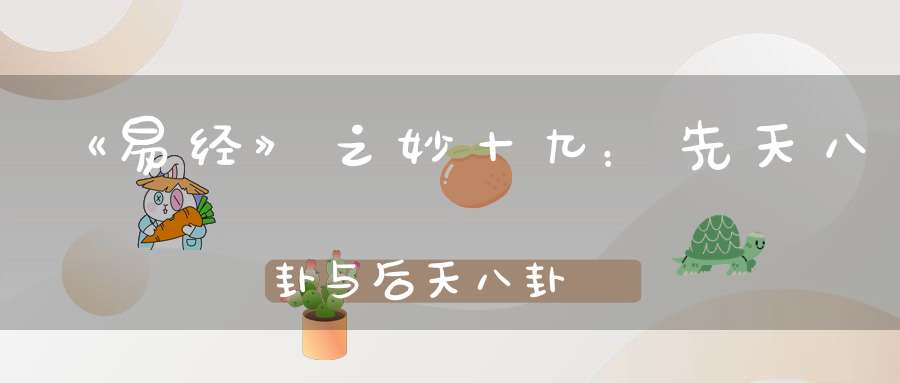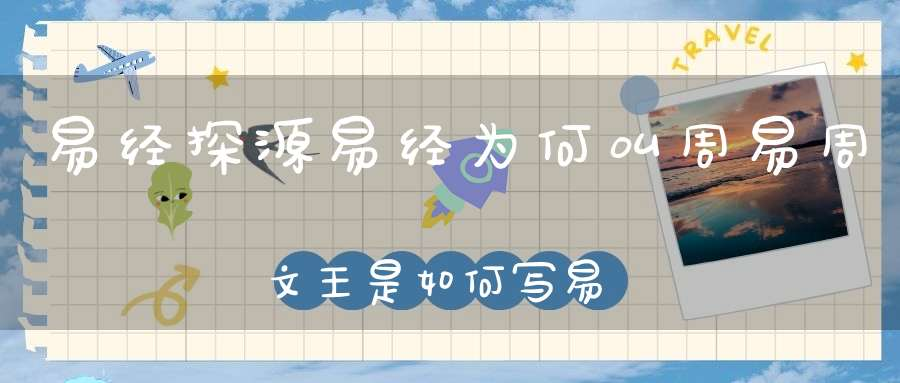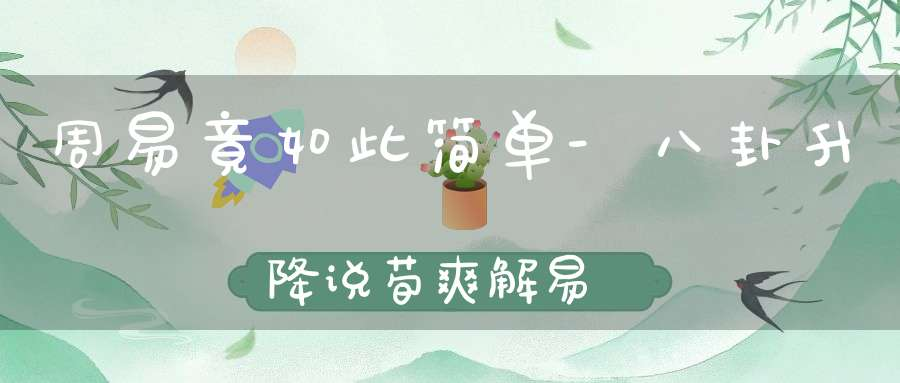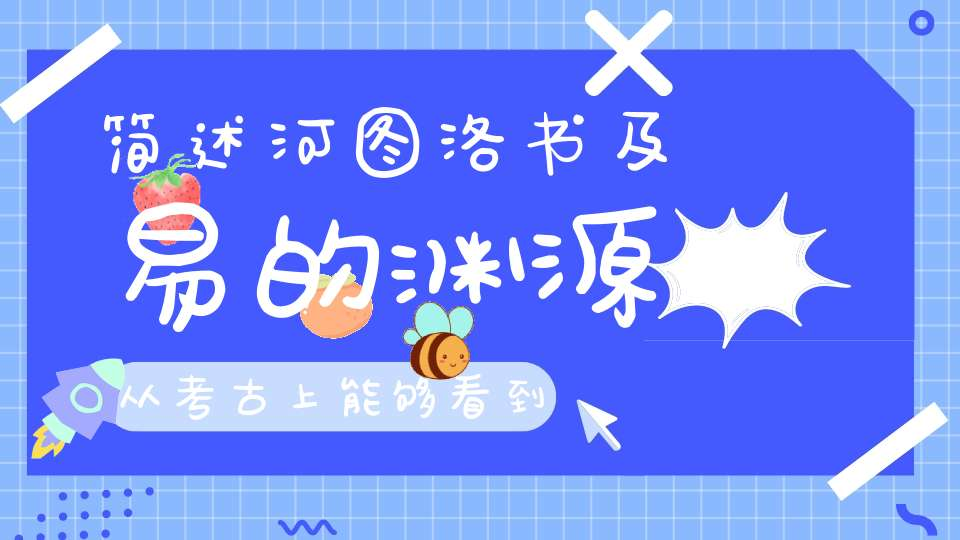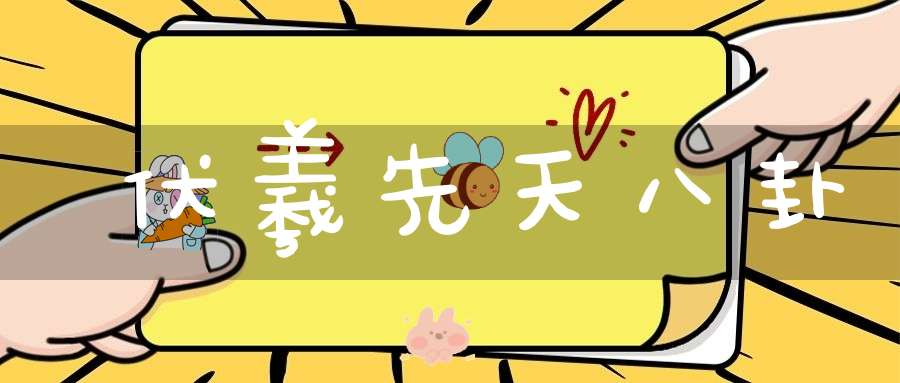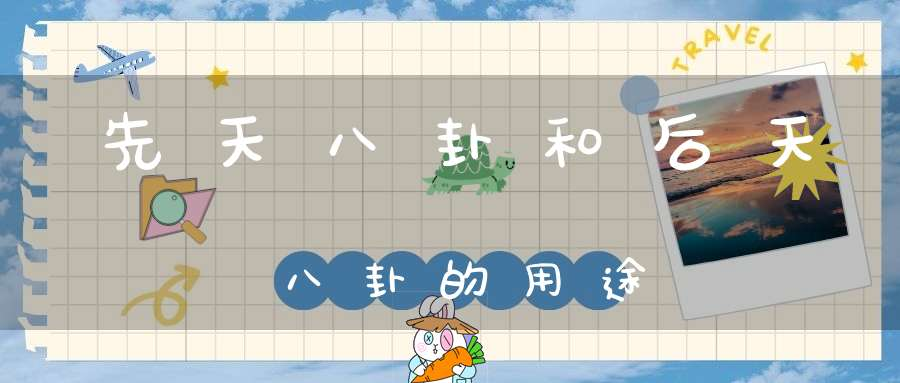登录

# 解读洛书九宫图数学奥秘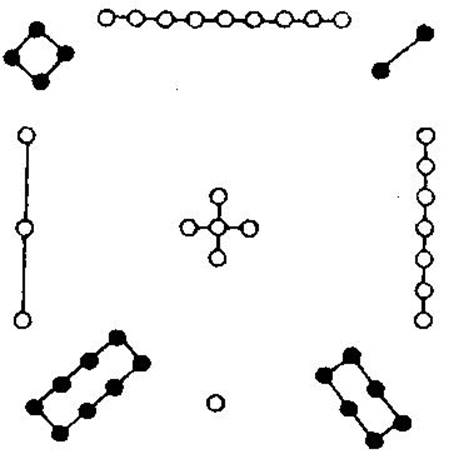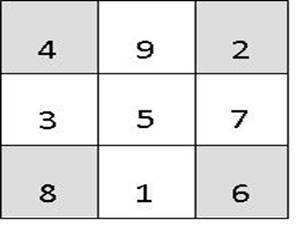1）当把这三个数两两循环组合，形成三个两位数相加时，数值和依然相等。具体操作如下：

49+92+24=35+57+73=81+16+68=165

29+94+42=75+53+37=61+18+86=165

2）当把这三个数三三循环组合，形成三个三位数相加时，数值和依然相等。具体操作如下：

492+924+249=357+573+735=816+168+681=1665

294+942+429=753+537+375=618+186+861=1665

3）当把三个数四四循环组合，形成三个四位数相加时，数值和依然相等。具体操作如下：

4924+9249+2492=3573+5735+7357=8168+1681+6816=16665

2942+9429+4294=7537+5375+3753=6186+1861+8618=16665

(1×2×3+4×5×6+7×8×9)-(1×4×7+2×5×8+3×6×9)=360